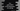# C++ program to print the ASCII values of all characters of a string## Introduction :

In this C++ tutorial, we will learn how to print the ASCII values of all characters of a string. Our program will take one string as an input from the user and print out the ASCII values of all characters.

The program will use one for loop to iterate through the string characters. Below, I will show you two different ways to iterate and print the ASCII of all characters.

## Example 1: Using iterator :

``````#include <iostream>
#include <string>
using namespace std;

int main(int argc, char const *argv[])
{
string givenString;
string::iterator iterator;

cout << "Enter a string : " << endl;
std::getline(std::cin, givenString);

for (iterator = givenString.begin(); iterator != givenString.end(); iterator++)
{
cout << *iterator << ": " << (int)*iterator << endl;
}

return 0;
}
``````

You can use one string iterator to iterate through the characters as shown above. It will print outputs as like below :

``````Enter a string :
hello
h: 104
e: 101
l: 108
l: 108
o: 111

Enter a string :
hello world
h: 104
e: 101
l: 108
l: 108
o: 111
: 32
w: 119
o: 111
r: 114
l: 108
d: 100
``````

## Example 2: Using range-based for loop in C++ 11 :

Range based for loop was introduced in C++ 11. It can iterate over a range of values.

``````#include <iostream>
#include <string>
using namespace std;

int main(int argc, char const *argv[])
{
string givenString;

cout << "Enter a string : " << endl;
std::getline(std::cin, givenString);

for (char const &ch : givenString)
{
cout << ch << ": " << (int)ch << endl;
}

return 0;
}
``````

We are iterating through the characters and printing the ASCII values one by one. It will print the same output as the above example.

``````Enter a string :
hello
h: 104
e: 101
l: 108
l: 108
o: 111
``````